# Introduction to Algorithms

## What's an Algorithm?

Definition - Algorithm: a set of instructions, or rules that, starting with initial data, solves a class of problems using precise operations, executed mechanically, without creative human intervention.

Cooking recipes could be thought of as algorithms. They enumerate a step-by-step procedure which achieves a task (the final dish) starting with initial data (ingredients) and asking you to execute relatively straight forward instructions. Yet, any of us who tried to use a cookbook know that 'straight forward' is such a relative term. Recipes tend to be ambiguous and could potentially be a nightmare for the beginner chef. So, then, what should we ask of a good recipe?

### Algorithms' properties

To qualify as an algorithm, the set of instructions should have some properties:

1. Preciseness - Or non-ambiguity. Each step must be non-ambiguous and executable without creative intervention.
2. Generality - It should solve a class of problems, not just one particular problem.
3. Finiteness - The algorithm must finish in a finite amount of time. For all practical purposes it should finish in reasonable time (e.g. before we die).
4. Correctness - The end result should be correct for all inputs.

Do recipes have the above properties? Supposedly they are correct, though some of your dinner guests might disagree (if they feed it to your dog while nobody's watching that might be a sign). They are also finite. What they lack is generality, since they cook one dish using fixed input. And what most of them lack is preciseness, which, on one hand makes them bad examples of algorithms, but on the other hand leaves way for creativity and uniqueness.

### Word's origins

The word algorithm has interesting roots. Some say it comes from al-Khwārizmī, a Persian mathematician who lived at around 800 and wrote books on linear and quadratic equations. Other people say it is an anagram of logarithm, a mathematical operation which is an inverse of the exponential. In the old days there seemed to be little distinction between algorithms and 'math recipes'. For a long time the word was linked with Euclid's algorithm for computing the greatest common divisor. See The timeline of algorithms for more details. Also, for a more detailed introduction to the concept visit Algorithm. We'll move on to see how we can create and describe algorithms.

## Flow Charts

Flow charts are one way to describe algorithms (another way is pseudocode, but we won't be dealing with that one here). They consist of the following symbols:

### Computation box

As the name suggests, these boxes are used to compute things. They usually comprise mathematical computations. Examples:

These boxes deal with the flow of data in and out of our algorithm. We can read (or input) initial values, as well as write (or output) the results. Examples:

### Decision box

A decision symbol is used to create a 'fork on the road'. It branches the execution depending on the result of the condition inside the box. If the expression is true, the execution of the algorithm continues on the right branch. If the expression is false the execution continues on the left branch. Examples:

We'll get a better idea about decision boxes when we design our first flow chart.

### Terminator box

I wouldn't even call this a proper symbol, we could probably do without it just fine. Its only role is to set where the algorithm starts and where it ends. Here are, for now, the two terminators we will use:

### Example one: Absolute value

We will now plunge into the hot waters of flow charts by designing our first one. And no, it's not the 'hello world' example, it's boring and so classical. Let us try to compute the following math function:$f(x)={\begin{cases}x,&{\mbox{if }}x\geq 0\\-x,&{\mbox{if }}x<0.\end{cases}}$

What function is this? Indeed, it's the absolute value, or modulus function. Let's build a flow chart that computes it:

To execute a flow chart, we start at the START symbol and follow the arrows. First thing we read x, the number for which we want to compute the absolute value. Then we test if it's less than zero. If so, we follow the right branch, the yes branch, where we compute y, the absolute value, as negative x. If x is greater or equal to zero than we follow the no branch, making x the value for y. Variable y holds the value of the function f(x). In the end we display y and we stop at the STOP symbol.

The flow chart implements the definition function exactly. One alternative is to display the result on each branch, without storing it in y (which means we don't really need y, we used it for extra clarity).

### Example two: Sum of the first n integers

Having successfully survived the encounter with our first algorithm, let's go on with a second one, shall we? Let's compute the following summation: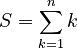$S=\sum _{{k=1}}^{n}k$

In human language that is the sum of the first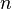$n$ integers: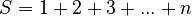$S=1+2+3+...+n$

Number$n$ is the input of our algorithm, while number$S$ is the output.

One way to do this is to have a counter, a variable that counts from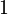$1$ to$n$; let's name it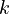$k$. More precisely,$k$ will hold all values from$1$ to$n$, one after another. For that, we create a loop in which$k$ is increased by$1$ on each iteration:

Remember, k ← k + 1 is interpreted as taking the old value of k, then adding 1 to it, and then storing it as the new value of k. Therefore, this flow chart section will add one, to k, in a loop. However, it will never end (and we know that is not quite an algorithm). To fix it, we have to add a condition to exit the loop when k exceeds$n$:

There is one more thing we need to do: our counter has to start somewhere. Let's initialize it with 1, the first number we need to add:

Now, that we have a counter which cycles through the numbers we need to add, all that's left to do is add it to our sum S. The sum needs to be initialized, as well. What should its initial value be? Math teaches us that zero is probably a good candidate, since it is the neutral value for addition.

The counter k has dual purpose, in our algorithm. First, it makes sure that the loop is executed n times. Second, it is used in computing the sum. In general, counters control the number of times the loop gets executed, but they don't always take part in the computation, as in our case.

The summation variable, S is an accumulator. It accumulates the result incrementally.

By this time I'd expect math geeks to jump up and down crying there's a simpler and more efficient way to do this. Indeed, there is a formula that computes the sum of the first n integers (derived from the general case of arithmetic progressions):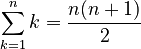$\sum _{{k=1}}^{n}k={\frac {n(n+1)}{2}}$

The flow chart for computing the sum becomes much simpler:

This is clearly a simpler and faster solution. There are two morals to this. First, we started with a more complicated solution to get our feet wet with a more complex example. Second, math is a powerful tool when building algorithms. Don't ignore it. Gauss certainly didn't. In elementary school his teacher, J.G. Büttner tried to occupy pupils by making them add up the integers from 1 to 100. The young Gauss produced the correct answer within seconds by a flash of mathematical insight, to the astonishment of all. Gauss had realized that pairwise addition of terms from opposite ends of the list yielded identical intermediate sums: 1 + 100 = 101, 2 + 99 = 101, 3 + 98 = 101, and so on, for a total sum of 50 × 101 = 5050. For more information, see Gauss' Biography and Gauss's Day of Reckoning.

To make everyone happy, including folks who might think "if he wanted a more complex example, why didn't he pick one that didn't have a trivial solution", imagine the problem of computing the product of the first$n$ integers, which mathematicians call the factorial:

Definition - Factorial: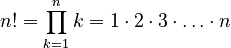$n!=\prod _{{k=1}}^{n}k=1\cdot 2\cdot 3\cdot \ldots \cdot n$

Not surprisingly, the algorithm looks the same as our first solution, except the + sign in S ← S + k becomes a * (* is the symbol for multiplication) and S has to be initialized to 1. There is no simple formula for computing n!.

This example introduces two common tools in algorithms: counters and accumulators. Let's try and define them.

Definition - Counter: a variable that keeps track of the number of times an event occurs. It is usually incremented by one at a time, and it is used frequently to count (and decide) the number of times a loop is executed.

Definition - Accumulator: a variable that holds a partial result and that aggregates values obtained incrementally along the computation process. The values are usually computed in a loop and they are usually added to the accumulator (though they could contribute in a more complex way). At the end of the process the accumulator contains the final result.

In other words, a counter counts, and an accumulator accumulates. What a surprise!

## Homework

Solve the following problems: First Degree Equation, Second Degree Equation.